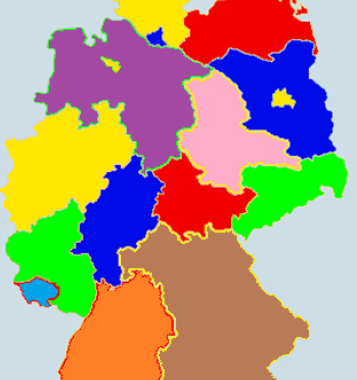# Map Coloring ProblemThis is a similar post as the one on Graph Coloring. However, this in this post I show an application of the graph coloring problem, allowing us to answer practical questions using graph theory. In this post we’ll first tackle a map coloring problem manually, then we’ll use a MiniZinc constraint programming script to validate the solution found.

### Our Challenge

Given is a map as shown beneath and the question is, how many colors we need at least to color this map, if we do not want neighboring states to be colored in the same color:

This problem can be transferred to a graph-based model. We consider the states to be nodes and the edges representing the neighborhood relationship between two states. An edge would express, that two connected nodes share a common border.

Instead of coloring the map, we can now solve the node coloring problem in the graph.

### Node Coloring Heuristic

To manually develop a solution, we could apply the following heuristic:

1. Choose a vertex with the highest grade and color it. Color as many vertices as possible with this color without coloring connected vertices with the same color.
2. Choose a new color and proceed with step 1 until all uncolored vertices are colored.

If following this heuristic, we would probably end up with something like this:

Based on the cycles L-K-F-L, M-J-O-M etc., we won’t find a solution with fewer than three colors. However, it is not immediately clear, that no solution is possible in this case with fewer than 4 colors and that the chromatic number χ(G) of the graph is equal to 4. From the 4 color theorem we know, that every map can be colored with no more than 4 colors.

### Validation using Constraint Programming

However, now we want to make sure, that no better coloring with fewer than four colors exists. For this purpose we transfer the problem to a constraint programming problem using the following script in MiniZinc:

``````int: nc = 3;      % max number of colors allowed

var 1..nc: a;     % define color variable for node a ...
var 1..nc: b;
var 1..nc: c;
var 1..nc: d;
var 1..nc: e;
var 1..nc: f;
var 1..nc: g;
var 1..nc: h;
var 1..nc: i;
var 1..nc: j;
var 1..nc: k;
var 1..nc: l;
var 1..nc: m;
var 1..nc: n;
var 1..nc: o;
var 1..nc: p;

constraint a != b;
constraint a != c;
constraint a != e;
constraint b != e;
constraint c != e;
constraint c != f;
constraint c != h;
constraint d != e;
constraint e != f;
constraint e != i;
constraint e != j;
constraint e != k;
constraint f != h;
constraint f != k;
constraint f != l;
constraint g != h;
constraint h != l;
constraint i != j;
constraint i != m;
constraint j != k;
constraint j != m;
constraint j != o;
constraint j != p;
constraint k != l;
constraint k != p;
constraint m != n;
constraint m != o;
constraint o != p;

solve satisfy;                  % solve model for satisfyability

output ["a=\(a)\t b=\(b)\t c=\(c)\n",       % plot results of variables
"d=\(d)\t e=\(e)\t f=\(f)\n",
"g=\(g)\t h=\(h)\t i=\(i)\n",
"j=\(j)\t k=\(k)\t l=\(l)\t",
"m=\(m)\t n=\(n)\t o=\(o)\t",
"p=\(p)\n"];``````

After running this script, we get the following output:

``````Running untitled_model.mzn
221msec

a=2	 b=3	 c=3
d=2	 e=1	 f=2
g=2	 h=1	 i=3
j=2	 k=3	 l=4	m=1	 n=2	 o=3	p=1
----------
Finished in 221msec.
``````

We see, that no 3-coloring is possible for this graph. After allowing 4 colors by setting int: nc = 4, a solution will be found:

``````Running untitled_model.mzn
215msec

a=2	 b=3	 c=3
d=2	 e=1	 f=2
g=2	 h=1	 i=3
j=2	 k=3	 l=4	m=1	 n=2	 o=3	p=1
----------
Finished in 215msec.
``````

Categories: